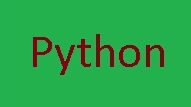# PYTHON FLOW CONTROL : QUESTION BANKQuestions for Practice:

1.What is Flow Chart ? Give the meaning of different flow chart symbols.

2.Write a Flow chart and Python program for the following:
a. To find the average of two numbers.
b. To find the simple interest given the value of P,T and R
c. To find the maximum of two numbers
d. To find the sum of first 50 natural numbers
e. To find the factorial of a given number N.

3.Compare == and = operator.

4.What are operators? Explain the following Operators with example:
a. Binary Boolean Operators: and, or & not

5.What is Flow Control ? Explain the different Elements of Flow Control?

6.Define Block and explain what nested blocks with example are.

7.Define condition control statement. Explain the different types of Condition Control Statement.

8.Explain with flow chart and programming example , the following condition control statements :
a. if
b. if – else
c. nested if else

9.What is iteration or looping ? Describe the different types of looping statements.

10.Explain with syntax , flow chart and programming example the following looping operations.
a. While loop
b. For loop

11.Discuss the working of range() function with programming example.

12.Give the output of the following :
a. range(10)
b. range(1,11)
c. range(0,30,5)
d. range(0,-9,-1)

13.What is infinite loop ? Explain with example.

14.What are nested Loops ? Explain with examples.

15.Discuss the following with examples :
a. break
b. continue
c. else statement with loop
d. pass

16.What are Python Modules ? Explain with examples how to import Python Modules .

17.What is the difference between break and continue statements.

18.What is the purpose of else in loop?

19.Write logical expressions for the following :
a. Either A is greater than B or A is less than C
b. Name is Snehith and age is between 18 and 35.
c. Place is either Mysore or Bengaluru but not “Dharwad”.

20.Convert the following while loop into for loop :
x =10
while (x<20):
print(x+10)
x+=2

21.Explain while and for loop . Write a program to generate Fibonacci series upto the given limit by defining FIBONACCI(n) function.

22.Mention the advantage of continue statement. Write a program to compute only even numbers sum within the given natural number using continue statement.

23.Demonstrate the use of break and continue keywords in looping structures using a snippet code.

24.With syntax explain the finite and infinite looping constructs in python. What is the break and continue statements .

Programming Questions for Practice:

1.Construct a logical expressions to represent each of the following conditions :
a. Mark is greater than or equal to 100 but less than 70
b. Num is between 0 and 5 but not equal to 2
c. Answer is either ‘N’ or ‘n’
d. Age is greater than or equal to 18 and gender is male
e. City is either ‘Kolkata’ or ‘Mumbai’

2.Write a program to check if the number of positive or negative and display an appropriate message.

3.Write a program to convert temperature in Fahrenheit to Celsius.

4.Write a program to display even numbers between 10 and 20.

5.Write a program to perform all the mathematical operations of calculator.

6.Write a program to accept a number and display the factorial of that number.

7.Write a program to convert binary number to decimal number.

8.Write a program to find the sum of the digits of a number.

9.Write a program to display prime number between 30.

10.Write a program to find the best of two test average marks out of three tests marks accepted from the user.

11.Write a program to find the largest of three numbers .[ VTU June /July 2019]

12.Write a program to check whether the given year is leap or not. [ VTU June /July 2019]

13.Write a program to generate and print prime number between 2 to 50.

14.Write a program to find those numbers which are divisible by 7 and multiple of 5 , between 1000 and 3000.

15.Write a program to guess a number between 1 to 10.

16.Write a program that accepts a word from the user and reverse it.

17.Write a program to count the number of even and odd numbers from a series of numbers.

18.Write a program that prints all the numbers from 0 to 10 except 3, 7 and 10.

19.Write a program to generate Fibonacci series between 0 and 50.

20.Write a program which iterates the integers from 1 to 50. For multiple of three print “Fizz” instead of the numbers and fro the multiples of five print “Buzz” . For numbers which are multiples of both three and five print “FizzBuzz”.

21.Write a program that accepts a string and calculate the number of digits and letters.

22.Write a program to check the validity of password input by users.

23.Write a program to find the numbers between 100 and 400 where each digit of a number is an even number . The numbers obtained should be printed in a comma-separated sequence.

24.Write a program to print alphabet patterns ‘A’ ,D, ‘E’, ‘G’,’L’, ‘T’ and ‘S’ with * symbol.

25.Write a python program to create the multiplication table (from 1 to 20) of a number.

26.Write a program to print the following patterns :

1
22
333
4444
55555
666666
7777777
88888888
999999999

1
1 2
1 2 3
1 2 3 4
1 2 3 4 5

A
B B
C CC
D DDD
E EEEE

1 2 3 4 5
1 2 3 4
1 2 3
1 2
1

*
* * *
* * * * *

1
2 3 2
3 4 5 4 3
4 5 6 7 6 5 4
5 6 7 8 9 8 7 6 5

``````* * * * *
* * *
*

1
1   1
1   2   1
1   3   3    1``````

1 4 6 4 1
1 5 10 10 5 1

1
2 3
4 5 6
7 8 9 10